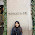## Wednesday, February 26, 2014

### 100 Questions

100 Questions

 12 + 9 =21 12 + 10 =22 3 + 11 =14 24 ÷ 4 =6 24 ÷ 6 =4 40 ÷ 8 =5 6 x 1 =6 56 ÷ 7 =8 0 + 6 =6 20 ÷ 5 =4 14 - 7 =7 16 ÷ 4 =4 7 x 6 =42 16 - 10 =6 13 - 6 =7 11 - 1 =10 1 + 2 =3 72 ÷ 9 =8 7 x 7 =47 1 + 2 =3 6 + 10 =16 3 x 0 =0 5 + 12 =17 4 + 7 =11 18 ÷ 9 =2 2 x 6 =12 1 x 8 =8 11 + 12 =23 12 - 2 =10 5 + 4 =9 14 ÷ 7 =2 4 x 6 =24 3 + 9 =12 8 - 1 =7 7 + 7 =14 8 + 9 =17 21 ÷ 3 =7 9 - 4 =5 9 x 1 =9 10 - 1 =9 5 + 5 =10 3 x 8 =24 12 + 8 =20 12 - 8 =4 11 - 7 =18 2 x 5 =10 0 + 7 =7 9 x 4 =36 45 ÷ 9 =5 11 - 3 =8 12 - 8 =4 14 - 5 =9 8 x 7 =56 4 + 8 =12 72 ÷ 9 =8 12 - 9 =3 42 ÷ 7 =6 2 + 0 =2 5 x 5 =25 9 - 3 =6 15 - 9 =6 11 - 7 =4 5 x 4 =20 4 ÷ 1 =4 24 ÷ 4 =6 30 ÷ 5 =6 9 x 5 =45 3 x 3 =9 3 ÷ 3 =1 16 ÷ 8 =2 15 - 9 =6 5 x 4 =20 14 ÷ 2 =7 2 ÷ 2 =1 4 + 8 =12 0 x 1 =0 12 - 4 =8 24 ÷ 8 =3 20 ÷ 5 =4 19 - 9 =10 72 ÷ 9 =8 4 x 6 =24 9 + 5 =14 64 ÷ 8 =8 1 x 7 =7 10 - 10 =0 1 x 9 =9 3 ÷ 3 =1 54 ÷ 6 =9 13 - 3 =10 8 x 5 =40 36 ÷ 6 =6 11 + 11 =22 This is 100 questions I did if you can do this sometime I well give you some hard one's 1 x 0 =0 13 - 4 =9 11 - 7 =4

1.2.